# Capital Cost

## Capital Cost

### Capital Cost

Chapter 15 The Cost of Capital

1.         Objectives

1.1       Explain the relationship between risk and return in financial investment.
1.2       Explain the nature and features of different securities in relation to the risk/return trade-off
1.3       Calculate cost of equity using dividend valuation model (DVM).
1.4       Calculate dividend growth using the dividend growth model.
1.5       Discuss the weaknesses of the DVM.
1.6       Define and distinguish between systematic and unsystematic risk.
1.7       Explain the relationship between systematic risk and return and describe the assumptions and components of the capital asset price model (CAPM).
1.9       Calculate the weighted average cost of capital (WACC) using book value and market value weightings.
1.10     Distinguish between average and marginal cost of capital.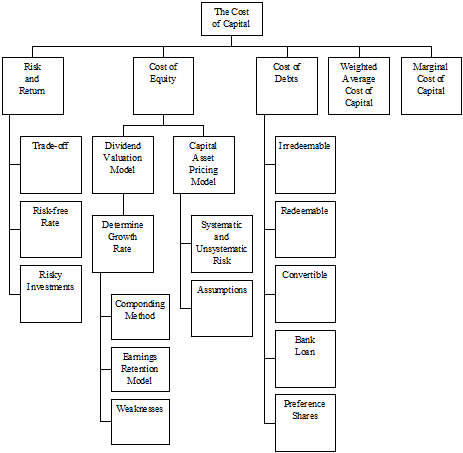2.         The Cost of Capital

 2.1 Cost of Capital (a)        The cost of capital is the rate of return that the enterprise must pay to satisfy the providers of funds, and it reflects the riskiness of providing funds. (b)        The cost of capital is an opportunity cost of finance, because it is the minimum return that investors require. If they do not get this return, they will transfer some or all of their investment somewhere else. Here are two examples: (i)        If a bank offers to lend money to a company, the interest rate it charges is the yield that the bank wants to receive from investing in the company, because it can get just as good a return from lending the money to someone else. In other words, the interest rate is the opportunity cost of lending for the bank. (ii)       When shareholders invest in a company, the returns that they can expect must be sufficient to persuade them not to sell some or all of their shares and invest the money somewhere else. The yield on the shares is therefore the opportunity cost to the shareholders of not investing somewhere else.

2.2       The cost of capital has two aspects to it.
(a)        The cost of funds that a company raises and uses, and the return that investors expect to be paid for putting funds into the company.
(b)       It is therefore the minimum return that a company should make on its own investment, to earn the cash flows out of which investors can be paid their return.
2.3       The cost of capital can therefore be measured by studying the returns required by investors, and then used to derive a discount rate for DCF analysis and investment appraisal.

(A)      The relationship between risk and return

2.4       When considering the return investors require, the trade-off with risk is of fundamental importance. Risk refers not to the possibility of total loss, but to the likelihood of actual return varying from those forecast.
2.5       Consider four investments opportunities: A, B, C and D shown on the risk/return chart below where:

The risk of project A = the risk of project B
The return from B = the return from C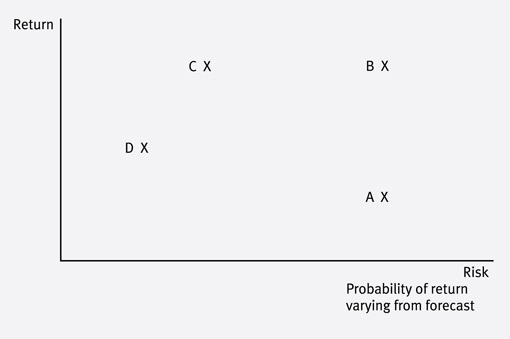In choosing between the investment opportunities:
B is preferable to A – higher return for the same risk.
C is preferable to B – same return for lower risk.
The choice between D and C is less clear-cut. C pays higher returns but this is to compensate for the comparatively higher associated risk. The choice will therefore depend on the investor’s attitude to risk and whether the increased return is seen by them as sufficient compensation for the higher level of risk.

(B)       The risk-free rate of return (Rf)

2.6       The Rf is the minimum rate required by all investors for an investment whose returns are certain. It is given in questions as:
(a)        the return on Treasury bills or
(b)        the return on government gilts (英國公債).
2.7       Once funds have been advanced to a company, an investor faces the risk that they will not be returned. However some investments are less risky than others. For example lending to a government is considered to be extremely low risk as governments are always able to raise funds via taxation to pay back the investor.
2.8       The risk is so minimal that government securities are known as risk-free and the return they pay is a minimum benchmark against which all other investments can be measured.
2.9       The return is sometimes given in examination questions as the return on Treasury Bills or gilts (gilt-edged securities).
(Gilt-edged securities (金邊證券) – 專指財政部代表政府發行的國家公債，由國家財政信譽作擔保，信譽度非常高。)

(C)      Return on risky investments – loan notes

2.10     Loan notes are lower risk investments than equities because the return is more predictable. This is because:
(a)        interest is a legal commitment
(b)        interest will be paid before any dividends
(c)        loans are often secured.
2.11     If a company issues loan notes, the returns needed to attract investors will therefore be:
(a)        higher than the Rf
(b)        lower than the return on equities.
2.12     Not all bonds have the same risk. There is a bond-rating system, which helps investors distinguish a company’s credit risk. Below is the Fitch and Standard & Poor’s bond rating scales.

 Fitch/S&P Grade Risk AAA Investment Highest quality AA Investment High quality A Investment Strong BBB Investment Medium grade BB, B Junk Speculative CCC/CC/C Junk Highly speculative D Junk In default

2.13     If the company falls below a certain credit rating, its grade changes from investment quality to junk status. Junk bonds are aptly named: they are the debt of companies in some sort of financial difficulty. Because they are so risky they have to offer much higher yields than other debt. This brings up an important point: not all bonds are inherently safer than shares.
2.14     The minimum investment grade rating is BBB. Institutional investors may not like such a low rating. Indeed some will not invest below an A rating.

(D)      Return on risky investments – equities

2.15     Equity shareholders are paid only after all other commitments have been met. They are the last investors to be paid out of company profits.
2.16     As their earnings also fluctuate, equity shareholders therefore face the greatest risk of all investors. The level of risk depends on:
(a)        volatility of company earnings
(b)        extent of other binding financial commitments.
2.17     The return required to entice investors into risky securities can be shown as

 Required return = Risk-free return + Risk premium

2.18     Since ordinary shares are the most risky investments the company offer, they are also the most expensive form of finance for the company.

3.         Estimating the Cost of Equity – Dividend Valuation Model

3.1      The cost of equity finance to the company is the return the investors expect to achieve on their shares.
3.2       New funds from equity shareholders are obtained either from new issues of shares or from retained earnings. Both of these sources of funds have a cost.
(a)        Shareholders will not be prepared to provide funds for a new issue of shares unless the return on their investment is sufficiently attractive.
(b)        Retained earnings also have cost. This is an opportunity cost, the dividend forgone by shareholders.

(A)      The dividend valuation model (DVM)

3.3       If we begin by ignoring share issue costs, the cost of equity, both for new issues and retained earnings, could be estimated by means of a dividend valuation model, on the assumption that the market value of shares is directly related to expected future dividends on the shares.
3.4       If the future dividend per share is expected to be constant in amount, then the ex dividend share price will be calculated by the formula: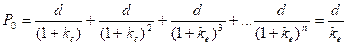So,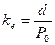Where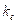is the cost of equity capital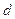is the annual dividend per share, starting at year 1 and then continuing annually in perpetuity.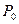is the ex-dividend share price

 3.5 Example 1 ABC Co has a dividend cover ratio of 4.0 times and expect zero growth in dividends. The company has one million \$1 ordinary shares in issue and the market capitalization (value) of the company is \$50 million. After-tax profits for next year are expected to be \$20 million. What is the cost of equity capital? Solution: Total dividends = 20 million/4 = \$5 million.= 5/50 = 10%

(B)       The dividend growth model

3.6       Shareholders will normally expect dividends to increase year by year and not to remain constant in perpetuity. The fundamental theory of share values states that the market price of a share is the present value of the discounted future cash flows of revenues from the share, so the market value given an expected constant growth in dividends would be: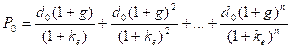Whereis the current market price (ex div)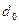is the current net dividendis the cost of equity capital
g is the expected annual growth in dividend payments
and bothand g are expressed as proportions.

It is often convenient to assume a constant expected dividend growth rate in perpetuity. The formula above then simplifies to: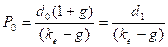Re-arrange this, we get a formula for the ordinary shareholders’ cost of capital.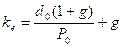or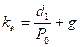3.7 Example 2 A share has a current market value of 96c, and the last dividend was 12c. If the expected annual growth rate of dividends is 4%, calculate the cost of equity capital. Solution: Cost of capital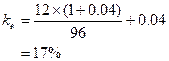(C)      Estimating the growth rate

(a)       Extrapolating based on past dividend patterns

3.8       This method assumes that the past pattern of dividends is a fair indicator of the future.

3.9

Example 3

 Year Dividends Earnings \$ \$ 2006 150,000 400,000 2007 192,000 510,000 2008 206,000 550,000 2009 245,000 650,000 2010 262,350 700,000

Dividends have risen from \$150,000 in 2006 to \$262,350 in 2010. The increase represents four years growth. (Check that you can see that there are four years growth, and not five years growth, in the table.)

The average growth rate, g, may be calculated as follows.
150,000 x (1 + g)4 = 262,350
g = 0.15 or 15%

The growth rate over the last four years is assumed to be expected by shareholders into the indefinite future. If the company is financed by equity and there are 1,000,000 shares in issue, each with a market value of \$3.35 ex div, the cost of equity,, is: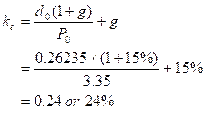(b)       Earnings retention model (Gordon’s growth model)

3.10     This model assumes that the higher level of retentions in a business, the higher the potential growth rate. The formula is therefore:

 g = bre Where re = accounting rate of return or ROCE or ROI b = earnings retention rate

 3.11 Example 4 A company is about to pay an ordinary dividend of 16c a share. The share price is 200c. The accounting rate of return on equity is 12.5% and 20% of earnings are paid out as dividends. Calculate the cost of equity for the company. Solution: b = 1 – dividend payout = 1 – 0.2 = 0.8 g = r x b = 0.125 x 0.8 = 0.1 P0 ex div = 200 – 16 = 184 d0 = 16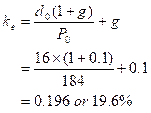3.12     Weaknesses of the dividend growth model
(a)        The model does not incorporate risk.
(b)        Dividend do not grow smoothly in reality so g is only an approximation.
(c)        The model fails to take capital gains into account, however it is argued that a change of share ownership does not affect the present value of the dividend stream.
(d)        No allowance is made for the effects of taxation although the model can be modified to incorporate tax.
(e)        It assumes there are no issue costs for new shares.

4.         Estimating the Cost of Equity – The Capital Asset Pricing Model (CAPM)

4.1       The CAPM can be used to calculate a cost of equity and incorporates risk. The CAPM is based on a comparison of the systematic risk of individual investments with the risks of all shares in the market.

(A)       Diversification

4.2      An investor, knowing that a particular investment was risky, could decide to reduce the overall risk faced, by acquiring a second share with a different risk profile and so obtain a smoother average return. Reducing the risk in this way is known as diversification.

For example, an investor is not confined to a pure investment in either Ace’s shares or Bravo’s shares. Another possibility is to buy a portfolio (投資組合), in other words, to split the fund between the two companies.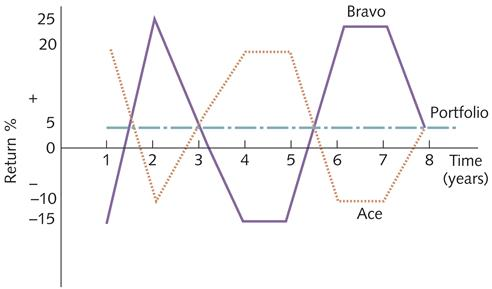4.3       Initial diversification will bring about substantial risk reduction as additional investments are added to the portfolio. However, risk reduction slows and eventually stops altogether once 15-20 carefully selected investments have been combined. This is because the total risk faced is not all of the same type.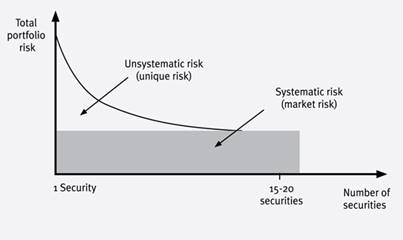4.4 Systematic and Unsystematic Risk The risk a shareholder faces is in large part due to the volatility of the company’s earnings. This volatility can occur because of: (a)      Systematic (or market) risk – it is the risk of market wide factors such as the state of economy. It will affect all companies in the same way (although to varying degrees), and it cannot be diversified away. (b)      Non-systematic (or unsystematic or business or unique) risk – it is the risk factors will impact each firm differently, depending on their circumstances. Diversification can almost eliminate unsystematic risk.
 4.5 Test your understanding 1 The following factors have impacted the volatility of the earnings of ABC Co, a manufacturer of chocolate biscuits and cereals: (a)      increase in interest rates (b)      increase in the price of cocoa beans (c)      legislation changing the rules on tax relief for investments in non-current assets (d)      growth in the economy of the country where ABC Co is based (e)      government advice on the importance of eating breakfast (f)       industrial unrest in ABC Co’s main factory. Are they examples of systematic or unsystematic risk?
 Factors Type of risk (a)      increase in interest rates (b)      increase in the price of cocoa beans (c)      legislation changing the rules on tax relief for investments in non-current assets (d)      growth in the economy of the country where ABC Co is based (e)      government advice on the importance of eating breakfast (f)       industrial unrest in ABC Co’s main factory.

(B)       Systematic risk and CAPM

 4.6 Beta Factor (a)      The CAPM is mainly concerned with how systematic risk is measured, and how systematic risk affects required return and share prices. Systematic risk is measured using beta factors. (b)      Beta factor is the measure of the systematic risk of a security relative to the market portfolio (e.g. Heng Seng Index). If a share price were to rise or fall at double the market rate, it would have a beta factor of 2.0. Conversely, if the share price moved at half the market rate, the beta factor would be 0.5. (c)      Beta = 1, a 1% change in the market index return generally leads to a 1% change in the return on a specific share. 0 < Beta < 1, a 1% change in the market index return generally leads to a less than a 1% change in the returns on a specific share. Beta > 1, a 1% change in the market index return generally leads to a greater than 1% on a specific company’s share.

4.7       A statistical analysis of historic returns from a security and from the average market may suggest that a linear relationship can be assumed to exist between them. A series of comparative figures could be prepared (month by month) of the return from a company’s shares and the average return of the market as a whole. The results could be drawn on a scatter graph and a “line of best fit” drawn (using linear regression techniques) as shown in the following figure.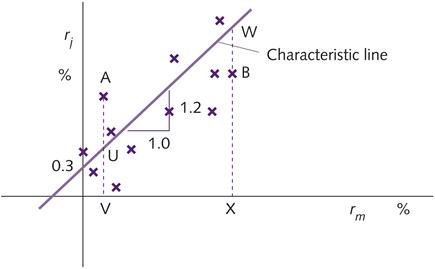4.8       The required return of a rational risk-averse well-diversified investor can therefore be found by returning to our original argument:

Required return = risk-free return + risk premium

 4.9 The CAPM Formula Required return: (Rj) = Rf + β(Rm - Rf) Where: Rj is the cost of equity capital Rf is the risk-free rate return Rm is the return from the market as a whole β is the beta factor of the individual security
 4.10 Example 5 Shares in ABC Co have a beta of 0.9. The expected returns to the market are 10% and the risk-free return is 4%. What is the cost of equity capital for ABC Co? Solution: Rj = Rf + β(Rm - Rf) = 4% + 0.9 x (10% – 4%) = 9.4%
 4.11 Test your understanding 2 Investors have an expected rate of return of 8% from ordinary shares in ABC Co, which have a beta of 1.2. The expected returns to the market are 7%. What will be the expected rate of return from ordinary shares in BBC Co, which have a beta of 1.8?

(C)       Assumptions and problems with applying the CAPM

 4.12 CAPM Assumptions (a)      Well-diversified investors (b)      Perfect capital market (c)      Unrestricted borrowing or lending at the risk-free rate of interest (d)      Uniformity of investor expectations (e)      All forecasts are made in the context of one time period only.
 4.13 Problems of CAPM Problems of CAPM include unrealistic assumptions and the required estimates being difficult to make. (a)      The need to determine the excess return (Rm - Rf). Expected, rather than historical, returns should be used, although historical returns are often used in practice. (b)      The need to determine the risk-free rate. A risk-free investment might be a government security. However, interest rates vary with the term of the lending. (c)      Errors in the statistical analysis used to calculate beta values. Betas may also change over time. (d)      The CAPM is also unable to forecast accurately returns for companies with low price/earnings ratios and to take account of seasonal “month-of-the-year” effects and “day-of-the-week” effects that appear to influence returns on shares.

5.         The Cost of Debt

5.1       Types of debt: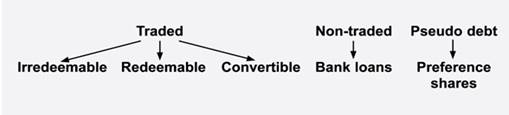5.2 Terminology (a)      The term loan notes, bonds, loan stock and marketable debt, are used interchangeably. Gilts are debts issued by the government. (b)      Irredeemable debt – no repayment of principal – interest in perpetuity. (c)      Redeemable debt – interest paid until redemption of principal. (d)      Convertible debt – may be later converted to equity. (e)      Vanilla bond (傳統債券，最普通的債券) – A bond with no unusual features, paying a fixed rate of interest and redeemable in full on maturity. The term derives from vanilla or 'plain' flavoured ice-cream.

5.3       It should be noted that different types of debt have different costs. The cost of a loan note will not be the same as the cost of a bank loan.

(A)       Irredeemable debt

 5.3 Formula Cost of irredeemable debt capital, paying interest i in perpetuity, and having a current ex-interest price P0: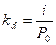5.4 Example 6 ABC Co has issued loan stock of \$100 nominal value with annual interest of 9% per year, based on the nominal value. The current market price of the loan stock is \$90. What is the cost of the loan stock? Solution: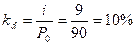5.5       If interest on loan capital is paid other than annually,

Cost of loan capital =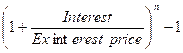Where n = number of times interest is paid per year

 5.6 Example 7 Henry has 12% irredeemable debentures in issue with a nominal value of \$100. The market price is \$95 ex interest. Calculate the cost of capital if interest is paid half-yearly. Solution: It interest is 12% annually, therefore 6% is payable half-yearly. Cost of loan capital =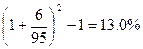(B)       Redeemable debt

5.7       If the debt is redeemable then in the year of redemption the interest payment will be received by the holder as well as the amount payable on redemption, so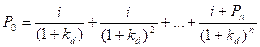Where Pn = the amount payable on redemption in year n.

5.8       The above equation cannot be simplified, so “r” will have to be calculated by trial and error, as an internal rate of return (IRR).

5.9

Example 8

Owen has in issue 10% loan notes of a nominal value of \$100. The market price is \$90 ex interest. Calculate the cost of this capital if the debenture is:

(a)      Irredeemable
(b)      Redeemable at par after 10 years
Ignore taxation

Solution:

(a)      The cost of irredeemable debt capital is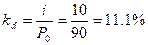(b)      The cost of redeemable debt capital. The capital profit that will be made from now to the date of redemption is \$10 (\$100 – \$90). This profit will be made over a period of ten years which gives an annualized profit of \$1 which is about 1% of current market value. The best trial and error figure to try first is therefore 12%.

 Year Cash flow (\$) DF 12% PV (\$) DF 11% PV (\$) 0 Market value (90) 1.000 (90.00) 1.000 (90.00) 1 – 10 Interest 10 5.650 56.5 5.889 58.89 10 Capital payment 100 0.322 32.2 0.352 35.20 (1.30) 4.09

The approximate cost of redeemable debt capital is, therefore: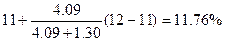(C)       Debt capital and taxation

5.10     The interest on debt capital is likely to be an allowable deduction for purposes of taxation and so the cost of debt and the cost of share capital are not properly comparable costs. This tax relief on interest ought to be recognized in computations. The after-tax cost of irredeemable debt is: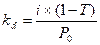5.11

Example 9

A company has in issue 12% redeemable debt with 5 years to redemption. Redemption is at par. The current market value of the debt is \$107.59. The corporate rate is 30%.

What is the cost of debt?

Solution:

 Year Cash flow (\$) DF 10% PV (\$) DF 5% PV (\$) 0 Market value (107.59) 1.000 (107.59) 1.000 (107.59) 1 – 5 Interest [12 x (1 – 30%)] 8.4 3.791 31.84 4.329 36.36 5 Capital payment 100 0.621 62.1 0.784 78.4 (13.65) 7.17

The approximate cost of redeemable debt capital is, therefore: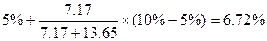(D)       Convertible debt

5.12     Convertible bonds carry a rate of interest in the same way as vanilla bonds, but they also give the holder the right to exchange the bonds at some stage in the future into ordinary shares according to some prearranged formula.
5.13     The owner of these bonds is not obliged to exercise this right of conversion and so the bonds may continue until redemption as interest-bearing instruments.

 5.14 Terminology (a)      Floor value – market value without the conversion option. (b)     Conversion ratio – this gives the number of ordinary shares into which a convertible bond may be converted: Conversion ratio =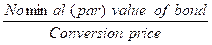(c)      Conversion price – this gives the price of each ordinary share obtainable by exchange a convertible bond: Conversion price =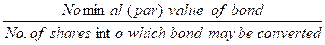(d)     Conversion premium – this gives the difference between the conversion price and the market share price, expressed as a percentage: Conversion premium =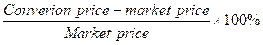(e)      Conversion value – this is the value of a convertible bond if it were converted into ordinary shares at the current share price: Conversion value = Current share price x Conversion ratio
 5.15 Example 10 A company has issued \$60 million 15-year 8.5% coupon bonds with a par value of \$100. Each bond is convertible into 40 shares of the company ordinary shares, which are currently trading at \$1.90. Required: (a)      What is the conversion price? (b)     What is the conversion premium? (c)      What is the conversion value of the bond? Solution: (a)      Conversion price = 100/40 = \$2.50 (b)     Conversion premium = (2.50 – 1.90)/1.90 = 31.6% (c)      Conversion value = \$1.90 x 40 = \$76
 5.16 Test your understanding 3 A company has issued convertible loan notes which are due to be redeemed at a 5% premium in five year’s time. The coupon rate is 8% and the current market value is \$85. Instead of the redemption payment, the investor can choose to convert each loan note into 20 shares on the same date. The company pays tax at 30% per annum. The company’s shares are currently worth \$4 and their value is expected to grow at a rate of 7% pa. Find the cost of the convertible debt to the company.

5.17     Bank and other non-tradeable fixed interest loans simply need to be adjusted for tax relief:

Cost to the company = Interest rate x (1 – T) [T = tax rate]

 5.18 Example 11 A firm has a fixed rate bank loan of \$1 million. It is charged 11% pa. The corporation tax rate is 30%. What is the cost of the loan? Solution: Cost of the loan = 11% x (1 – 30%) = 7.7%

(F)       Preference shares

5.19     Although not strictly debt, the fixed rate of dividend and the fact that they are paid before ordinary shareholders, mean that preference shares are often treated as a form of lending similar to irredeemable debentures.
5.20     The main difference between preference shares and debt is that the preference dividend payments are not tax deductible. The formulae are therefore: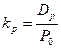Where Dp = the constant annual preference dividend
P0 = ex div market value of the share

 5.21 Example 12 A company has 50,000 8% preference shares in issue, nominal value \$1. The current ex div market value is \$1.20 per share. What is the cost of the preference shares? Solution: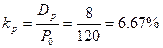6.         The Weighted Average Cost of Capital (WACC)

6.1       In the analysis so far carried out, each source of finance has been examined in isolation. However, the practical business situation is that there is a continuous raising of funds from various sources.
6.2       These funds are used, partly in existing operations and partly to finance new projects. These is not normally any separation between funds from different sources and their application to specific projects: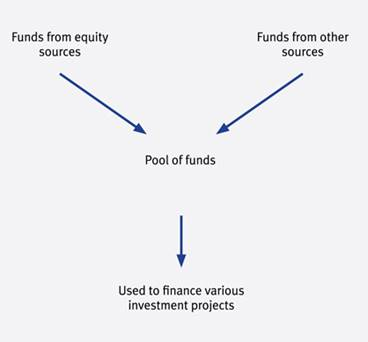6.3       Even if a question tells you that a project is to be financed by the raising of a particular loan or through an issue of shares, in practice the funds raised will still be added to the firm’s pool of funds and it is from that pool that the project will be funded.
6.4       It is therefore not the marginal cost of the additional finance, but the overall average cost of all finance raised, that is required for project appraisal.
6.5       The general approach is to calculate the cost of each individual source of medium-long term finance and then weight it according to its importance in the financing mix.

 6.6 WACC (a)      Weighted average cost of capital is the average cost of the company’s finance (equity, debentures, bank loans) weighted according to the proportion each element bears to the total pool of capital. (b)     A general formula for the WACC is as follows.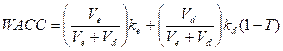Where ke = the cost of equity kd = the cost of debt Ve = the market value of equity in the firm Vd = the market value of debt in the firm T = the rate of company tax (c)       To find an average cost, the various sources of finance must be weighted according to the amount of each held by the company. The weights for the sources of finance could be: Book values – represents historic cost of finance. Market values – represent current opportunity cost of finance. Wherever possible market value should be used because book values based on historical costs and their use will seriously understate the impact of the cost of equity finance. If the WACC is underestimated, unprofitable projects will be accepted.

6.7

Example 13

A entity has the following information in its statement of financial position.

 \$000 Ordinary shares of 50c 2,500 12% unsecured loan notes 1,000

The ordinary shares are currently quoted at 130c each and the loan notes are trading at \$72 per \$100 nominal. The ordinary dividend of 15c has just been paid with an expected growth rate of 10%. Corporation tax is currently 30%.

Calculate the WACC for this entity.

Solution:

Market values:

 \$000 Equity (Ve): 2,500/0.5 x 1.30 6,500 Loan notes (Vd): 1,000 x 0.72 720 7,220

Cost of equity: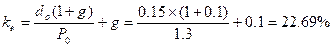Cost of loan stock: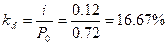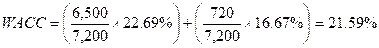7.         Marginal Cost of Capital (MCC)

 7.1 MCC MCC is the cost associated with raising one additional dollar of capital. It can be argued that the current weighted average cost of capital should be used to evaluate projects. Where a company’s capital structure changes only very slowly over time, the marginal cost of new capital should be roughly equal to the weighted average cost of current capital. Where gearing levels fluctuate significantly, or the finance for new project carries a significantly different level of risks to that of the existing company, there is good reason to seek an alternative marginal cost of capital.

7.2

Example 14

A company has the following capital structure:

 After tax cost Market value After tax cost x Market value Source % \$m Equity 12 10 1.2 Preference 10 2 0.2 Loan notes 7.5 8 0.6 20 2.0

WACC =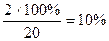The company’s directors have decided to embark on major capital expenditure, which will be financed by a major issue of funds. The estimated project cost is \$3,000,000, 1/3 of which will be financed by equity, 2/3 of which will be financed by loan notes. As a result of undertaking the project, the cost of equity (existing and new shares) will rise from 12% to 14%. The cost of preference shares and the cost of existing loan notes will remain the same, while the after tax cost of the new loan notes will be 9%.

Required:

Calculate the company’s new WACC, and its marginal cost of capital.

Solution:

New WACC

 After tax cost Market value After tax cost x Market value Source % \$m Equity 14 11 1.54 Preference 10 2 0.2 Existing loan notes 7.5 8 0.60 New loan notes 9 2 0.18 23 2.52

WACC =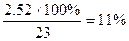MCC =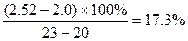Examination Style Questions

Question 1
Burse Co wishes to calculate its weighted average cost of capital and the following information relates to the company at the current time:

 Number of ordinary shares 20 million Book value of 7% convertible debt \$29 million Book value of 8% bank loan \$2 million Market price of ordinary shares \$5.50 per share Market value of convertible debt \$107.11 per \$100 bond Equity beta of Burse Co 1.2 Risk-free rate of return 4.7% Equity risk premium 6.5% Rate of taxation 30%

Burse Co expects share prices to rise in the future at an average rate of 6% per year. The convertible debt can be redeemed at par in eight years’ time, or converted in six years’ time into 15 shares of Burse Co per \$100 bond.

Required:

(a)     Calculate the market value weighted average cost of capital of Burse Co. State clearly any assumptions that you make.                                                                                        (12 marks)
(b)     Discuss the circumstances under which the weighted average cost of capital can be used in investment appraisal.                                                                                                   (6 marks)
(c)     Discuss whether the dividend growth model or the capital asset pricing model offers the better estimate of the cost of equity of a company.                                                           (7 marks)
(Total 25 marks)
(ACCA F9 Financial Management June 2008 Q1)

Question 2
Rupab Co is a manufacturing company that wishes to evaluate an investment in new production machinery. The machinery would enable the company to satisfy increasing demand for existing products and the investment is not expected to lead to any change in the existing level of business risk of Rupab Co.

The machinery will cost \$2.5 million, payable at the start of the first year of operation, and is not expected to have any scrap value. Annual before-tax net cash flows of \$680,000 per year would be generated by the investment in each of the five years of its expected operating life. These net cash inflows are before taking account of expected inflation of 3% per year. Initial investment of \$240,000 in working capital would also be required, followed by incremental annual investment to maintain the purchasing power of working capital.

Rupab Co has in issue five million shares with a market value of \$3.81 per share. The equity beta of the company is 1.2. The yield on short-term government debt is 4.5% per year and the equity risk premium is approximately 5% per year.

The debt finance of Rupab Co consists of bonds with a total book value of \$2 million. These bonds pay annual interest before tax of 7%. The par value and market value of each bond is \$100.

Rupab Co pays taxation one year in arrears at an annual rate of 25%. Capital allowances (tax-allowable depreciation) on machinery are on a straight-line basis over the life of the asset.

Required:

(a)     Calculate the after-tax weighted average cost of capital of Rupab Co.   (6 marks)
(b)     Prepare a forecast of the annual after-tax cash flows of the investment in nominal terms, and calculate and comment on its net present value.                                                            (8 marks)
(c)     Explain how the capital asset pricing model can be used to calculate a project-specific discount rate and discuss the limitations of using the capital asset pricing model in investment appraisal.       (11 marks)
(Total 25 marks)
(ACCA F9 Financial Management December 2008 Q3)

Question 3
CAP is a listed company that owns and operates a large number of farms throughout the world. A variety of crops are grown.

Financing structure
The following is an extract from the balance sheet of CAP at 31 March 2010.

 \$m Ordinary shares of \$1 each 200 Reserves 100 9% irredeemable \$1 preference shares 50 8% loan stock 2011 250 600

The ordinary shares were quoted at \$3 per share ex div on 31 March 2010. The beta of CAP’s equity shares is 0.8, the annual yield on treasury bills is 5%, and financial markets expect an average annual return of 15% on the market index.

The market price per preference share was \$0.90 ex div on 31 March 2010.

Lock stock interest is paid annually in arrears and is allowable for tax at a tax rate of 30%. The loan stock was priced at \$100.57 ex interest per \$100 nominal on 31 March 2010. The loan stock is redeemable on 31 March 2011.

Assume that taxation is payable at the end of the year in which taxable profits arise.

A new project
Difficult trading conditions in US farming have caused CAP to decide to convert a number of its farms in Southern American into camping sites with effect from the 2011 holiday season. Providing the necessary facilities fro campers will require major investment, and this will be financed by a new issue of loan stock. The returns on the new campsite business are likely to have a very low correlation with those of the existing farming business.

Required:

(a)     Using the capital asset pricing model, calculate the required rate of return on equity of CAP at 31 March 2010. Ignore any impact from the new campsite project. Briefly explain the implications of a Beta of less than 1, such as that for CAP.                                                                     (4 marks)
(b)     Calculate the weighted average cost of capital of CAP at 31 March 2010 (use your calculation in answer to requirement (a) above for the cost of equity). Ignore any impact from the new campsite project.                                                                                                                (10 marks)
(c)     Without further calculations, identify and explain the factors that may change CAP’s equity beta during the year ending 31 March 2011.
(5 marks)
(d)     Explain the limitations of the capital asset pricing model.                       (6 marks)
(Total 25 marks)

Source: https://hkiaatevening.yolasite.com/resources/FMNotes/Chapter15-CostofCapital.doc

Web site to visit: https://hkiaatevening.yolasite.com

Author of the text: indicated on the source document of the above text

If you are the author of the text above and you not agree to share your knowledge for teaching, research, scholarship (for fair use as indicated in the United States copyrigh low) please send us an e-mail and we will remove your text quickly. Fair use is a limitation and exception to the exclusive right granted by copyright law to the author of a creative work. In United States copyright law, fair use is a doctrine that permits limited use of copyrighted material without acquiring permission from the rights holders. Examples of fair use include commentary, search engines, criticism, news reporting, research, teaching, library archiving and scholarship. It provides for the legal, unlicensed citation or incorporation of copyrighted material in another author's work under a four-factor balancing test. (source: http://en.wikipedia.org/wiki/Fair_use)

The information of medicine and health contained in the site are of a general nature and purpose which is purely informative and for this reason may not replace in any case, the council of a doctor or a qualified entity legally to the profession.

#### Capital Cost

The texts are the property of their respective authors and we thank them for giving us the opportunity to share for free to students, teachers and users of the Web their texts will used only for illustrative educational and scientific purposes only.

All the information in our site are given for nonprofit educational purposes

##### Capital Cost

Topics and Home
Contacts
Term of use, cookies e privacy